## Wednesday, November 19, 2008

### Learn how to develop a three tier web application using (Asp.net) with source code

This article focuses on explaining how to code a three tier asp.net web application visual basic. Here I will be explaining how you can bind an object to the data grid and display the data from the database just by adding the references to the business logic and data access dlls.

Ok first open a web project in visual studio 2005 and in Default.aspx add a Gridview and name it grdStudents

Now you need to add the references to the business tier dlls and data tier dlls.

Add the following references to the project:

ThreeTierVB.BusinessLogic.dll
ThreeTierVB.DataAccess.dll
ThreeTierVB.Info.dll

How to get these dlls is explained in detail in How to develop a three tier .net application using visual basic with source Code.

Now after adding the references to the project
copy and paste the following code in the code behind page (Default.aspx.vb) of your Default.aspx

Partial Class _Default
Inherits System.Web.UI.Page
Private _students As ThreeTierVB.Info.StudentInfo()

Protected Sub Page_Load(ByVal sender As Object, ByVal e As System.EventArgs) Handles Me.Load
BindGrid()
End Sub

Private Sub BindGrid()
Dim clsS As New ThreeTierVB.BusinessLogic.StudentsBLL
_students = clsS.GetStudentByClass(1)

With grdStudents

.DataSource = _students
.Columns.Item(0).Visible = False
.Columns.Item(3).Visible = False

.DataBind()
End With
End Sub
End Class

In the above code we have declared the collection of students and bind the collection of the students to the gridview grdStudents.

Now run the application and you can see the grid filled with data.

Now lets make the grid a bit preety by adding the following code in your default.aspx page:

just replace the grid view code with the following: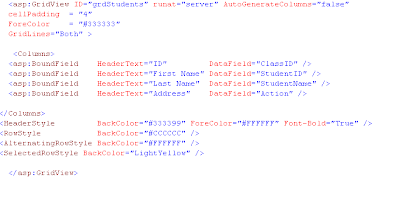Now run the application and see the new look of the grid.

Thus now you can code any three tier web or desktop applicaion in .net.

Read more!

## Thursday, November 6, 2008

### Learn how to develop a three tier .net application using (vb.net) with visual basic source code

This article focuses on explaining how to code a three tier application using
visual basic .net . Here I will be explaining each an every step from creating
new sql server database table to running the vb program going through most of
the vb code in the application and also providing some links that I find useful
for the learners.In this visual basic net tutorial, I will be mainly focusing
the beginners to mid level .net programmers so I will try to expalin each and
every detail as I can but even that if I missed something don't hesitate to post
a comment on this blog article. I hope this will be a good vb tutorial for
whoever is interested in it and this application can be easily extended to asp
net three tier application for web developers.

Now lets start (Note: Source code of this tutorial is available at the end of
this article)

Step 1

Let's first create two tables in the sql server database with the following sql
query:

Table 1 : Class

CREATE TABLE [dbo].[Class](
[ClassID] [int] IDENTITY(1,1) NOT NULL,[ClassName] [varchar](50) COLLATE SQL_Latin1_General_CP1_CI_AS NULL,
CONSTRAINT [PK_Class] PRIMARY KEY CLUSTERED
(
[ClassID] ASC) ON [PRIMARY]
) ON [PRIMARY]

(Note: You can create the tables yourself in Microsoft Sql Server Management
Studio and it looks like the image shown below)Table 2 : Students

CREATE TABLE [dbo].[Students](
[StudentID] [int] IDENTITY(1,1) NOT NULL,
[StudentName] [varchar](50) COLLATE SQL_Latin1_General_CP1_CI_AS NULL,
[ClassID] [int] NULL,
CONSTRAINT [PK_Students] PRIMARY KEY CLUSTERED
(
[StudentID] ASC
) ON [PRIMARY]
) ON [PRIMARY]

ALTER TABLE [dbo].[Students] WITH CHECK ADD CONSTRAINT [FK_Students_Class]

FOREIGN KEY([ClassID])
REFERENCES [dbo].[Class] ([ClassID])

(Note: You can create the tables yourself in Microsoft Sql Server Management
Studio and it looks like the image shown below)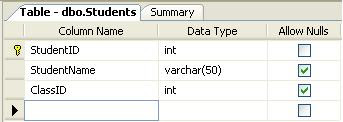Now here you have to create a Foreign key constraint to classID (If you don't
know what foreign key is then you can (Learn Foreign key)

Step 2

Now create a window project in visual basic and name it ThreeTierVB and save
your project and solution in your desired Path.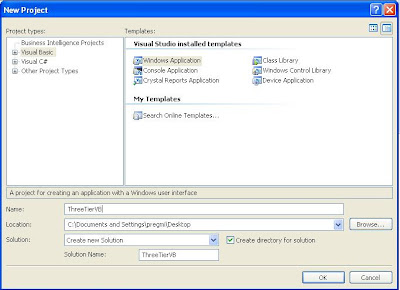Add Two folders in the project by right clicking the ThreeTierVB on your
solution explorer and
Add --> New Folder and name them
Forms
GlobalClasses

Right click the forms Folder and add a windows form and name it frmStudent then
add the following components in the form and name them accordingly as follows:

1 combobox
name:cboClass

4 command Buttons
name: cmdNew
Text: New Class

name: cmdClassSave
Text: Save Class

name: cmdSave
Text: Save

name: cmdExit
Text: Exit

2 text boxes
name: txtClassName

name: txtStudentName

1 Datagridview

name: grdStudents

A quick snapshot of my form is shown below:(add the labels and name the labels appropriately and also you can place the controls wherever you like them comfortable)

Step 3

Now before going to the coding lets create the three tier structure so that it
would be easier to understand for beginner programmers.

1. Add a new Class library to the solution by going to
File --> Add --> New project

2. Select the class library and name it ThreeTierVB.BusinessLogic and
add two folders BLLClasses and GenericClasses as explained on step 2

3. Similarly add another class library as above and name it ThreeTierVB.DataAccess
and add three folders and name them DataClasses, GlobalClasses and Modules

4. Similarly add another class library as above and name it ThreeTierVB.Info
and add a folder and name it InfoClasses

Now your solution Explorer should look like the following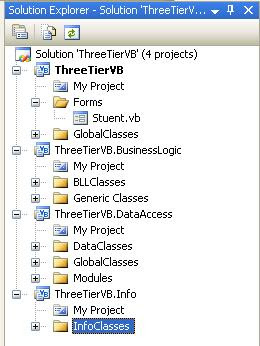Step 4

Now we have got 4 projects in our solution Explorer. Lets make the idea clear
Now here we are making DataAccess , BusinessLogic and GuI the three tiers and
Info is the info for all the three tiers, so we have to be clear on what get
access to what.

Add the references to the projects

1. ThreeTierVB which is our presentation tier and this needs to know the info
and business logic but it doesn't worry about Dataaccess.

For this right click on ThreeTierVB project and Addreference in the add reference screen go to projects and select ThreeTierVB.Businesslogic and ThreeTierVB.Info by clicking ctrl and click ok button.2. Similarly Businesslogic only needs to know the DataAccess and info so as done
in 1 add references to ThreeTierVB.DataAccess and ThreeTierVB.Info.

3. Now in DataAccess project just add ThreeTierVB.Info as a reference.

Step 5

Add the required classes in the projects to run the application now as we have
set up all the necessary references.

1. In ThreeTierVB.Info add two classes and name them as ClassInfo and StudentInfo in ClassInfo Write the following code:

Public Class ClassInfo

#Region "Private Member Declarations"

Private mClassId As Integer
Private mClassName As String

Private mAction As Byte

#End Region

#Region "Public Properties"

Public Property Action() As Byte
Get
Return mAction
End Get
Set(ByVal value As Byte)
mAction = value
End Set
End Property

Public Property ClassID() As Integer
Get
Return mClassId
End Get
Set(ByVal value As Integer)
mClassId = value
End Set
End Property

Public Property ClassName() As String
Get
Return mClassName
End Get
Set(ByVal value As String)
mClassName = value
End Set
End Property

#End Region

End Class

In StudentInfo Write the following code:

Public Class StudentInfo

#Region "Private Member Declarations"

Private mStudentID As Integer
Private mStudentName As String
Private mClassID As Integer

Private mAction As Byte

#End Region

#Region " Public Properties"

Public Property Action() As Byte
Get
Return mAction
End Get
Set(ByVal value As Byte)
mAction = value
End Set
End Property

Public Property StudentID() As Integer
Get
Return mStudentID
End Get
Set(ByVal value As Integer)
mStudentID = value
End Set
End Property

Public Property ClassID() As Integer
Get
Return mClassID
End Get
Set(ByVal value As Integer)
mClassID = value
End Set
End Property

Public Property StudentName() As String
Get
Return mStudentName
End Get
Set(ByVal value As String)
mStudentName = value
End Set
End Property
#End Region

End Class

These two classes will act as objects of student and class throughout the
solution.

Now your Solution Explorer will look like the following: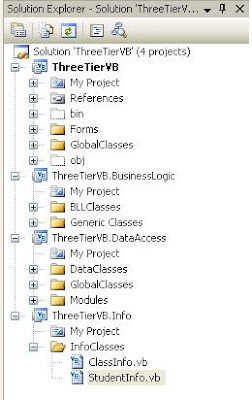2. In ThreeTierVB.DataAccess

In DataClasses folder add ClasDB and StudentsDB classes and in GlobalClasses
folder add sqlHelp class and add a module modDB in modules folder.
I will just provide the code below for this tutorial but for full details on how
to access data from the database you can check How to insert record in SQL
server database from VB.Net
?

Now in SQLHelp class add the following code:

Imports System.Data.SqlClient

Public NotInheritable Class SqlHelp

'Since this class provides only static methods, make the default constructor private to prevent
'instances from being created with "new SqlHelper()".

Dim conn As SqlConnection
Dim cmd As SqlCommand
Dim isTran As Boolean
Private trans As SqlTransaction
Dim connStr As String

Private Function Connect() As Boolean
Dim bln As Boolean
' Try
If conn Is Nothing Then
bln = ReadDatabseConfig(strDBConfFile)
If bln = True Then
conn = New SqlConnection(connStr)
End If
End If
If conn.State = ConnectionState.Closed Then
conn.Open()
End If

Exit Function

End Function

Public Sub BeginTransaction()

If isTran Then Return
If conn.State = ConnectionState.Closed Then
conn.Open()
End If
trans = conn.BeginTransaction()
isTran = True
End Sub

Public Sub CommitTransaction()
If Not isTran Then Return
trans.Commit()
conn.Close()
trans = Nothing
isTran = False
End Sub

Public Sub RollBackTransaction()
If Not isTran Then Return
trans.Rollback()
conn.Close()
trans = Nothing
isTran = False
End Sub

Public Sub CloseConn()
If Not conn Is Nothing Then
If Not conn.State = ConnectionState.Closed Then

conn.Close()
End If
End If
End Sub

Public Function ExecuteQuery(ByVal strCmdTxt As String) As Boolean
Dim intRows As Integer

If conn.State = ConnectionState.Closed Then
Connect()
End If

cmd = New SqlCommand
cmd.Connection = conn
cmd.CommandText = strCmdTxt
cmd.CommandType = CommandType.Text

If Not isTran Then
intRows = cmd.ExecuteNonQuery()
conn.Close()
Else
cmd.Transaction = trans
intRows = cmd.ExecuteNonQuery()

End If

If intRows> 0 Then
ExecuteQuery = True
Else
ExecuteQuery = False
End If

End Function

Public Function ExecuteAndGetID(ByVal strCmdTxt As String, Optional ByVal

blnNonID As Boolean = False) As String

If conn.State = ConnectionState.Closed Then
Connect()
End If
If Not blnNonID Then
strCmdTxt = strCmdTxt & " ; select scope_Identity();"
End If
cmd = New SqlCommand
cmd.Connection = conn
cmd.CommandText = strCmdTxt
cmd.CommandType = CommandType.Text

If Not isTran Then
ExecuteAndGetID = CStr(cmd.ExecuteScalar())
conn.Close()
Else
cmd.Transaction = trans
ExecuteAndGetID = CStr(cmd.ExecuteScalar())
End If

End Function

Public Function ExecuteAndGetReader(ByVal strCmdTxt As String) As

SqlDataReader

If conn.State = ConnectionState.Closed Then
Connect()
End If

cmd = New SqlCommand
cmd.Connection = conn
cmd.CommandText = strCmdTxt
cmd.CommandType = CommandType.Text

If Not isTran Then
ExecuteAndGetReader = cmd.ExecuteReader

Else
cmd.Transaction = trans
ExecuteAndGetReader = cmd.ExecuteReader
End If

End Function

Public Function ExecuteAndGetRow(ByVal strCmdTxt As String) As DataRow

Dim dt As DataTable
Dim da As SqlDataAdapter
Dim row As DataRow

If conn.State = ConnectionState.Closed Then
Connect()
End If
cmd = New SqlCommand
cmd.Connection = conn
cmd.CommandText = strCmdTxt
cmd.CommandType = CommandType.Text
dt = New DataTable
If Not isTran Then

da = New SqlDataAdapter(cmd)
Else
cmd.Transaction = trans
da = New SqlDataAdapter(cmd)
End If

da.Fill(dt)
da.Dispose()
row = dt.Rows(0)
ExecuteAndGetRow = row

End Function

Public Function getDataset(ByVal strCmdTxt As String) As DataSet
Dim da As SqlDataAdapter = New SqlDataAdapter
Dim ds As DataSet = New DataSet

If conn.State = ConnectionState.Closed Then

Connect()
End If

cmd = New SqlCommand
cmd.Connection = conn
cmd.CommandText = strCmdTxt
cmd.CommandType = CommandType.Text
If isTran Then
cmd.Transaction = trans
End If
da.SelectCommand = cmd
da.Fill(ds)
If Not isTran Then
conn.Close()
End If
Return ds

End Function

Public Sub New()

If Not Connect() Then
Exit Sub
End If
End Sub

Private Function ReadDatabseConfig(ByVal filename As String) As Boolean

Dim result As Boolean
Dim strDataSource As String = ""
Dim strInitialCatalog As String = ""
Dim strUserID As String = ""
Dim strPassword As String = ""

Dim sr As New IO.StreamReader(filename)
Dim ln As String = sr.ReadLine
While IsNothing(ln) = False
If ln.StartsWith("DataSource") = True Then
strDataSource = getConfigValue(ln)
ElseIf ln.StartsWith("DBaseName") = True Then
strInitialCatalog = getConfigValue(ln)
ElseIf ln.StartsWith("UserID") Then
strUserID = getConfigValue(ln)
ElseIf ln.StartsWith("Password") = True Then
strPassword = getConfigValue(ln)

End If
ln = sr.ReadLine
End While
sr.Close()
connStr = "Data Source=" & strDataSource & ";" & "Initial Catalog=" &

strInitialCatalog & _
";User ID=" & strUserID & ";Password=" & strPassword
result = True
ReadDatabseConfig = result

End Function

Private Function getConfigValue(ByVal line As String) As String
Dim values() As String = Split(line, "=")
Dim reply As String = values(1).Trim

Return reply
End Function
End Class

In Module modDB add the following code

Module modDB
Public strDBConfFile As String = "C:\DBConfig.ini"
Public Enum FlagAction
Insert = 1
Update = 2
Delete = 3
NoAction = 0
End Enum
End Module

Similarly in ClassDB add the following code

Imports System.Data.SqlClient
Imports ThreeTierVB.Info

Public Class classDB

' The function below will Delete the row of the class table with the provided
class ID. But to do this we have to first delete the related students in the
class because class has relation with the student table, this is the one of the
advantages of relational database.

Public Function DeleteClass(ByVal ClassID As Integer) As Boolean
Dim strSql As String
Dim objdb As New SqlHelp

strSql = "Delete from Students where ClassID= " & ClassID
objdb.ExecuteQuery(strSql)
objdb = New SqlHelp
strSql = "Delete from Class where ClassID= " & ClassID
objdb.ExecuteQuery(strSql)

Return True
End Function

' The function below gets all the rows of the class table and returns the
classInfo() collection so that this collection can be used in presentation
layer.

Public Function GetClass() As ClassInfo()
Dim strSql As String
Dim objdb As New SqlHelp

strSql = "Select * from Class"
Dim dr As SqlDataReader = objdb.ExecuteAndGetReader(strSql)
Dim arr As New ArrayList
While dr.Read

Dim cls As ClassInfo = New ClassInfo
cls.ClassID = IIf(IsDBNull(dr("ClassID")), 0, dr("ClassID"))
cls.ClassName = IIf(IsDBNull(dr("ClassName")), "", dr("ClassName"))
arr.Add(cls)
End While
dr.Close()
Return CType(arr.ToArray(GetType(ClassInfo)), ClassInfo())

End Function

'The function below gets the particular row of the class with the provided
classID and again returns the collection.

Public Function GetClass(ByVal ClassId As Integer) As ClassInfo()
Dim strSql As String
Dim objdb As New SqlHelp

strSql = "Select * from Class where classID= " & ClassId
Dim dr As SqlDataReader = objdb.ExecuteAndGetReader(strSql)
Dim arr As New ArrayList
While dr.Read

Dim cls As ClassInfo = New ClassInfo
cls.ClassID = IIf(IsDBNull(dr("ClassID")), 0, dr("ClassID"))
cls.ClassName = IIf(IsDBNull(dr("ClassName")), "", dr("ClassName"))
arr.Add(cls)
End While
dr.Close()
Return CType(arr.ToArray(GetType(ClassInfo)), ClassInfo())

End Function

' The below function gets the classInfo object as the parameter and saves the
record in the database and return true when inserted successfully.

Public Function Save(ByVal cls As ClassInfo) As Boolean
Dim strsql As String = ""
Dim objDB As New SqlHelp
If cls.Action = FlagAction.Insert Then
strsql = "Insert into Class (ClassName) " & _
"values( '" & cls.ClassName.Trim() & "')"
ElseIf cls.Action = FlagAction.Update Then
strsql = "Update Class set " & _
"ClassName='" & cls.ClassName.Trim() & "'" & _
" where ClassID=" & cls.ClassID
Else
Return False
End If
objDB.ExecuteQuery(strsql)
Return True
End Function

End Class

Similarly in StudentsDB add the following code

Imports System.Data.SqlClient
Imports ThreeTierVB.Info

Public Class StudentsDB

' The function deletes the student

Public Function DeleteStudent(ByVal StudentID As Integer) As Boolean
Dim strSql As String
Dim objDB As SqlHelp
strSql = "Delete from Students where StudentID=" & StudentID
objDB = New SqlHelp
objDB.ExecuteQuery(strSql)
Return True
End Function

'Retrieves the collection of student
Public Function GetStudent() As StudentInfo()
Dim objdb As New SqlHelp
Dim strSql As String = ""
strSql = "Select * from Students"
Dim dr As SqlDataReader = objdb.ExecuteAndGetReader(strSql)
Dim arr As New ArrayList
While dr.Read
Dim sI As StudentInfo = New StudentInfo
sI.ClassID = IIf(IsDBNull(dr("ClassID")), 0, dr("ClassID"))
sI.StudentID = IIf(IsDBNull(dr("StudentID")), 0, dr("StudentID"))
sI.StudentName = IIf(IsDBNull(dr("Student")), "", dr("StudentName"))
arr.Add(sI)
End While
dr.Close()
Return CType(arr.ToArray(GetType(StudentInfo)), StudentInfo())
End Function

'Retrieves the collection of student
Public Function GetStudent(ByVal StudentID As Integer) As StudentInfo()
Dim objdb As New SqlHelp
Dim strSql As String = ""
strSql = "Select * from Students where StudentID= " & StudentID
Dim dr As SqlDataReader = objdb.ExecuteAndGetReader(strSql)
Dim arr As New ArrayList
While dr.Read
Dim sI As StudentInfo = New StudentInfo
sI.ClassID = IIf(IsDBNull(dr("ClassID")), 0, dr("ClassID"))
sI.StudentID = IIf(IsDBNull(dr("StudentID")), 0, dr("StudentID"))
sI.StudentName = IIf(IsDBNull(dr("Student")), "", dr("StudentName"))
arr.Add(sI)
End While
dr.Close()
Return CType(arr.ToArray(GetType(StudentInfo)), StudentInfo())
End Function

'Retrieves the collection of student provided the classID as the parameter

Public Function GetStudentByClass(ByVal ClassID As Integer) As StudentInfo()
Dim objdb As New SqlHelp
Dim strSql As String = ""
strSql = "Select * from Students where ClassID= " & ClassID
Dim dr As SqlDataReader = objdb.ExecuteAndGetReader(strSql)
Dim arr As New ArrayList
While dr.Read
Dim sI As StudentInfo = New StudentInfo
sI.ClassID = IIf(IsDBNull(dr("ClassID")), 0, dr("ClassID"))
sI.StudentID = IIf(IsDBNull(dr("StudentID")), 0, dr("StudentID"))
sI.StudentName = IIf(IsDBNull(dr("StudentName")), "", dr

("StudentName"))
arr.Add(sI)
End While
dr.Close()
Return CType(arr.ToArray(GetType(StudentInfo)), StudentInfo())
End Function

' The function below saves the student

Public Function Save(ByVal student As StudentInfo) As Boolean
Dim strsql As String = ""
Dim objDB As New SqlHelp
If student.Action = FlagAction.Insert Then
strsql = "Insert into Students (StudentName,ClassID) " & _
"values( '" & student.StudentName.Trim() & "', " &

student.ClassID & ")"
ElseIf student.Action = FlagAction.Update Then
strsql = "Update Students set" & _
"StudentName='" & student.StudentName & "'," & _
" ClassID=" & student.ClassID & "' " & _
" where StudentID=" & student.StudentID
End If
objDB.ExecuteQuery(strsql)
Return True
End Function

End Class

3. In ThreeTierVB.BusinessLogic

In BLLClasses folder add classBll and studentsBll classes
and in classBLL add the following code:

Public Class classBLL
' This class now just acts as a bridge between the presentation layer and the
data access layer but later as the application grows most of the business cases
and business level logic can be implemented in this layer.

Public Function GetClass() As ThreeTierVB.Info.ClassInfo()
Dim db As New ThreeTierVB.DataAccess.classDB
Return db.GetClass()
End Function

Public Function GetClass(ByVal ClassID As Integer) As

ThreeTierVB.Info.ClassInfo()
Dim db As New ThreeTierVB.DataAccess.classDB
Return db.GetClass(ClassID)
End Function

Public Function Save(ByVal cls As ThreeTierVB.Info.ClassInfo)
Dim db As New ThreeTierVB.DataAccess.classDB
Return db.Save(cls)
End Function

Public Function Delete(ByVal intClassID As Integer)
Dim db As New ThreeTierVB.DataAccess.classDB
Return db.DeleteClass(intClassID)
End Function

End Class

Similarly in StudentBLL add the following code:

Public Class StudentsBLL
Public Function GetStudent() As ThreeTierVB.Info.StudentInfo()
Dim db As New ThreeTierVB.DataAccess.StudentsDB
Return db.GetStudent
End Function

Public Function GetStudent(ByVal studentID As Integer) As

ThreeTierVB.Info.StudentInfo()
Dim db As New ThreeTierVB.DataAccess.StudentsDB
Return db.GetStudent(studentID)
End Function

Public Function GetStudentByClass(ByVal intClassID As Integer)
Dim db As New ThreeTierVB.DataAccess.StudentsDB
Return db.GetStudentByClass(intClassID)
End Function

Public Function Save(ByVal student As ThreeTierVB.Info.StudentInfo)
Dim db As New ThreeTierVB.DataAccess.StudentsDB
Return db.Save(student)
End Function

Public Function Delete(ByVal intStudentID As Integer)
Dim db As New ThreeTierVB.DataAccess.StudentsDB
Return db.DeleteStudent(intStudentID)
End Function

End Class

Step 6 - Presentation Layer starts here

3. In ThreeTierVB

Now we have completed all the Business logic and Dataaccess coding and came back
to the form that we have left before.

Add the following code to the form: (Note if error you can copy individual
functions and subs and paste it in your form code page accordingly)

Public Enum FlagAction
Insert = 1
Update = 2
Delete = 3
NoAction = 0
End Enum

Public Class frmStudent

Private _students As ThreeTierVB.Info.StudentInfo()
Private _student As ThreeTierVB.Info.StudentInfo
Private _class As ThreeTierVB.Info.ClassInfo
Private _classes As ThreeTierVB.Info.ClassInfo()

Private Sub frmStudent_Load(ByVal sender As System.Object, ByVal e As
System.EventArgs) Handles MyBase.Load

LoadCbo()
LoadGrid(cboClass.SelectedValue)
End Sub

Sub LoadCbo()
' Get data for Class combo
Dim clsC As New ThreeTierVB.BusinessLogic.classBLL
_classes = clsC.GetClass
With cboClass

'to understand the following you can see How to use an object as a data source
in Visual Studio 2005
?
.DataSource = _classes
.DisplayMember = "ClassName"
.ValueMember = "ClassID"
End With
' cboClass.SelectedIndex = 0

End Sub

Sub LoadGrid(ByVal classID As Integer)
Dim clsS As New ThreeTierVB.BusinessLogic.StudentsBLL
_students = clsS.GetStudentByClass(classID)

With grdStudents

'to understand the following you can see How to use an object as a data
source in Visual Studio 2005
?
.DataSource = _students
.ColumnHeadersVisible = True

.ColumnHeadersDefaultCellStyle.ForeColor = Color.BurlyWood
.Columns.Item("ClassID").Visible = False
.Columns.Item("StudentID").HeaderText = "Student ID"
.Columns.Item("StudentName").HeaderText = "Student Name"
.Columns.Item("Action").Visible = False
End With
End Sub

Sub SaveClass()
Dim bln As Boolean
Dim clsC As New ThreeTierVB.BusinessLogic.classBLL
Dim clsInfo As New ThreeTierVB.Info.ClassInfo
clsInfo.ClassName = Me.txtClassName.Text.Trim
If cboClass.SelectedIndex = -1 Then
clsInfo.Action = FlagAction.Insert
Else
clsInfo.ClassID = cboClass.SelectedValue
clsInfo.Action = FlagAction.Update
End If
bln = clsC.Save(clsInfo)

If bln Then
MessageBox.Show("Data updated Successfully!!")
If cboClass.SelectedIndex = -1 Then
LoadCbo()
cboClass.SelectedIndex = UBound(_classes)
End If
_classes.SetValue(clsInfo, cboClass.SelectedIndex)

Refresh(cboClass.SelectedIndex)
grpNewClass.Visible = False
grpMain.Enabled = True
Else
MessageBox.Show("There is some error Updating record!! Try again")
End If
End Sub

Public Overloads Sub Refresh(ByVal intID As Integer)
cboClass.DataSource = _classes
cboClass.Refresh()
cboClass.SelectedIndex = intID

End Sub

Sub SaveStudent()
Dim bln As Boolean
Dim stuB As New ThreeTierVB.BusinessLogic.StudentsBLL
Dim stuInfo As New ThreeTierVB.Info.StudentInfo
Dim cls As ThreeTierVB.Info.ClassInfo
cls = _classes.GetValue(cboClass.SelectedIndex)
stuInfo.ClassID = cls.ClassID
stuInfo.StudentName = Me.txtStudentName.Text
stuInfo.Action = FlagAction.Insert
bln = stuB.Save(stuInfo)
MessageBox.Show("Student Data Updated Successfully!!")

End Sub

Private Sub cmdClassSave_Click(ByVal sender As System.Object, ByVal e As
System.EventArgs) Handles cmdClassSave.Click

SaveClass()
End Sub

Private Sub cboClass_SelectedIndexChanged(ByVal sender As System.Object,
ByVal e As System.EventArgs) Handles cboClass.SelectedIndexChanged

If cboClass.SelectedIndex = -1 Then Exit Sub
Dim cls As ThreeTierVB.Info.ClassInfo
grpNewClass.Text = "Change this Class Name"
cls = _classes.GetValue(cboClass.SelectedIndex)
LoadGrid(cls.ClassID)
Me.txtClassName.Text = cls.ClassName

End Sub

Private Sub cmdNew_Click(ByVal sender As System.Object, ByVal e As
System.EventArgs) Handles cmdNew.Click
Me.txtClassName.Text = ""
Me.cboClass.SelectedIndex = -1
grpNewClass.Text = "Add New Class Name"
grpNewClass.BackColor = Color.MintCream
grpNewClass.Visible = True
grpMain.Enabled = False

Me.txtClassName.Focus()
End Sub

Private Sub cmdSave_Click(ByVal sender As System.Object, ByVal e As
System.EventArgs) Handles cmdSave.Click
errDisplay.Clear()
If cboClass.SelectedIndex = -1 Then
errDisplay.SetError(Me.cboClass, "Please Select Class!!")
Exit Sub
End If
If Me.txtStudentName.Text.Trim() = "" Then
errDisplay.SetError(Me.txtStudentName, "Please Enter Student Name")

Exit Sub
End If
SaveStudent()

End Sub
Private Sub cmdExit_Click(ByVal sender As System.Object, ByVal e As

System.EventArgs) Handles cmdExit.Click
Application.Exit()
End Sub

End Class

Now you can run the application and test, if you find errors try debugging it as
enough information was provided during this tutorial. If not you can download
this full application by clicking the link provided below.

Note: for website developers the presentation layer can be your web page and you
can bind your data to the _Students or _classes collection objects as well.

Download full three tier vb.net sample application

Read more!